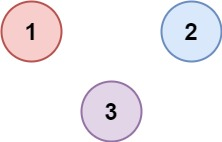# 547. Number of Provinces

There are `n` cities. Some of them are connected, while some are not. If city `a` is connected directly with city `b`, and city `b` is connected directly with city `c`, then city `a` is connected indirectly with city `c`.

province is a group of directly or indirectly connected cities and no other cities outside of the group.

You are given an `n x n` matrix `isConnected` where `isConnected[i][j] = 1` if the `ith` city and the `jth` city are directly connected, and `isConnected[i][j] = 0` otherwise.

Return the total number of provinces.

Example 1:`Input: isConnected = [[1,1,0],[1,1,0],[0,0,1]]`
```Output: 2
```

Example 2:`Input: isConnected = [[1,0,0],[0,1,0],[0,0,1]]`
```Output: 3
```

Constraints:

• `1 <= n <= 200`
• `n == isConnected.length`
• `n == isConnected[i].length`
• `isConnected[i][j]` is `1` or `0`.
• `isConnected[i][i] == 1`
• `isConnected[i][j] == isConnected[j][i]`